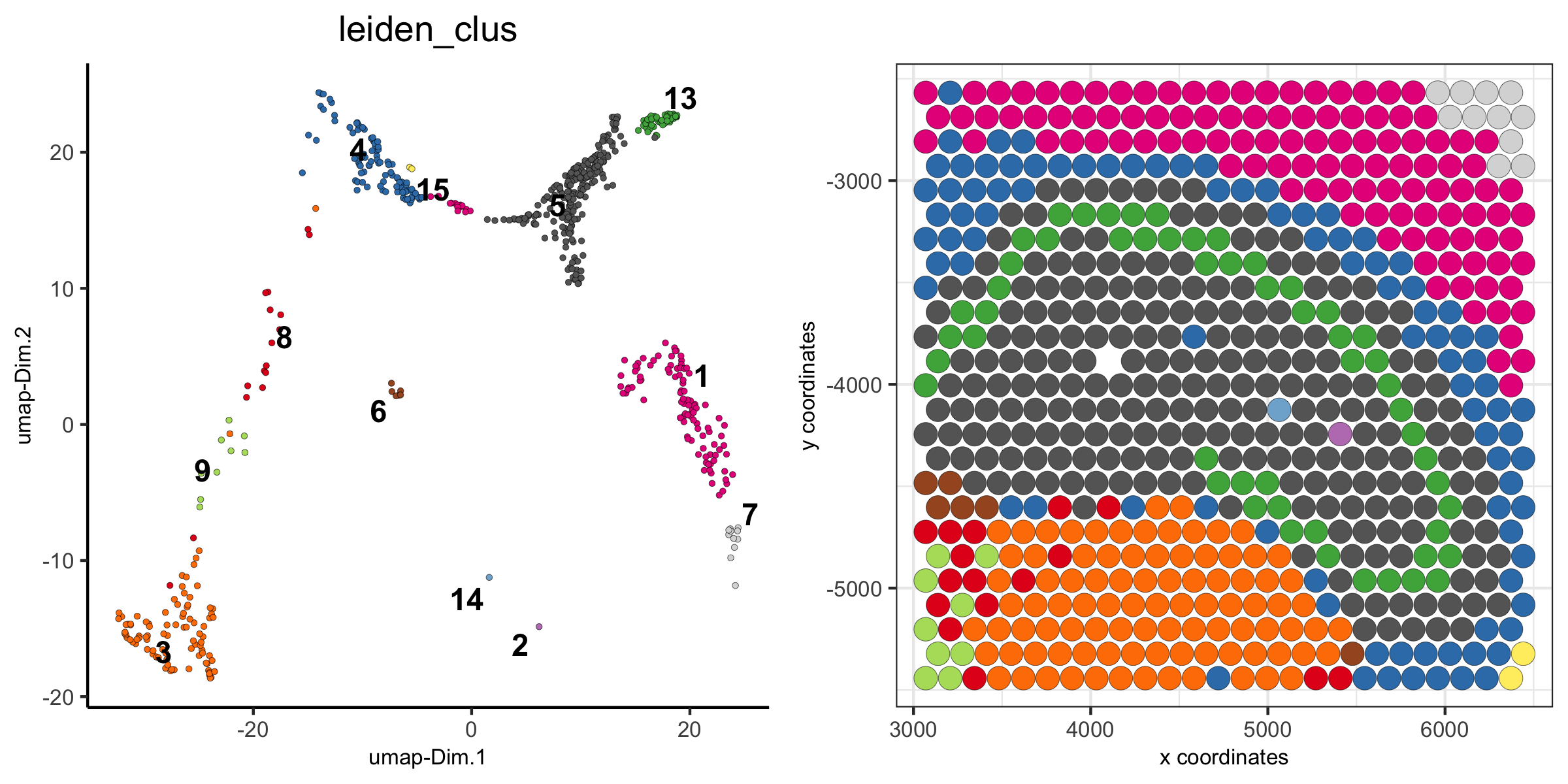# How to Subset a Giotto Object?¶

There are several ways to subset a Giotto object or visualize a subset of the data. Below are some subsetting examples.

Note

Figures may not be identical, because examples might have been updated, but the subsetting principles remain the same.

## 1. The Visium 10X Whole Brain¶

```spatDimPlot(visium_brain, cell_color = 'leiden_clus',
plot_alignment = 'horizontal', spat_point_size = 2)
```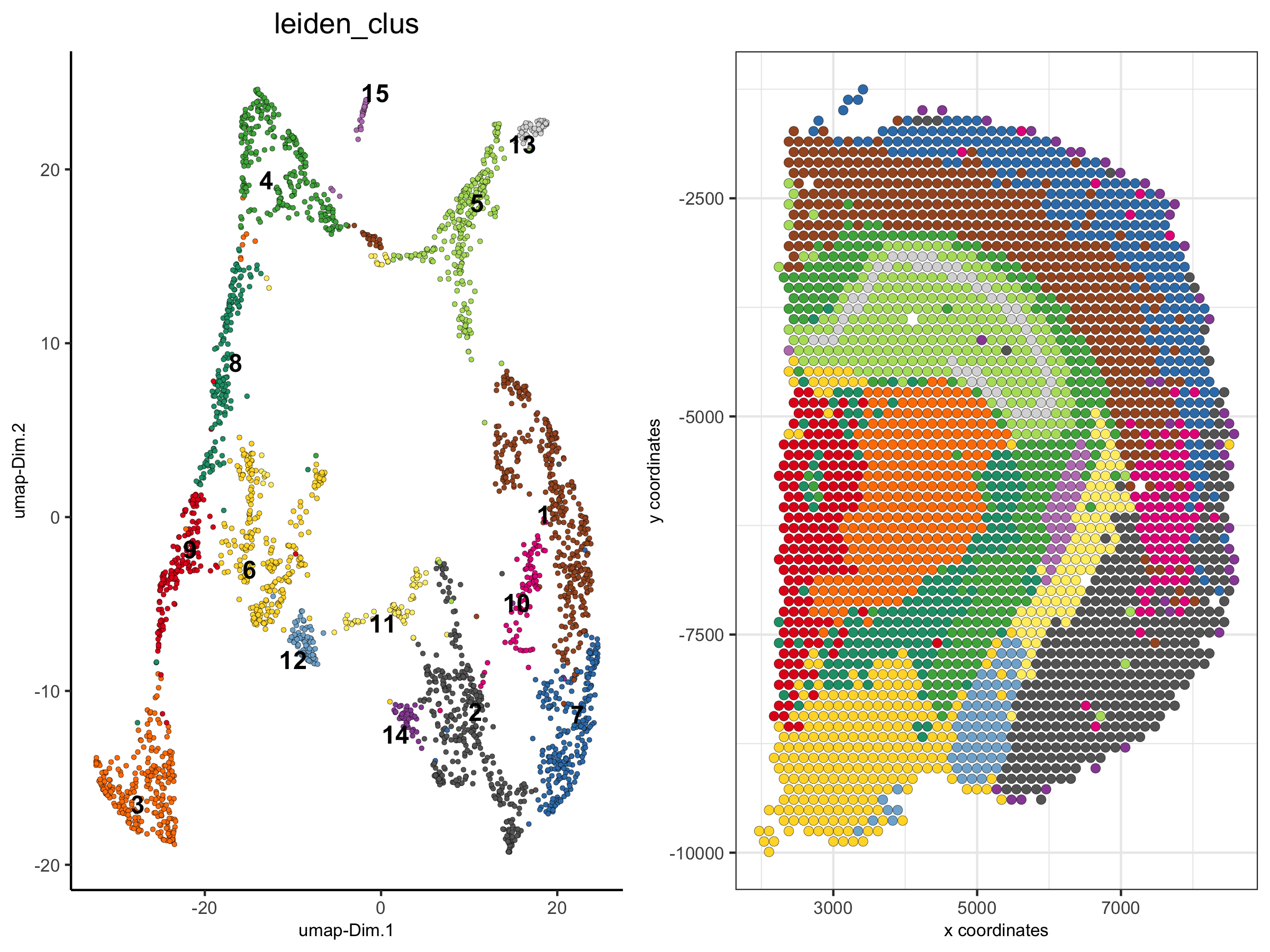## 2. Show Only A Subset of Clusters¶

```spatDimPlot(visium_brain,
cell_color = 'leiden_clus',  select_cell_groups = '4',
plot_alignment = 'horizontal', spat_point_size = 2)
```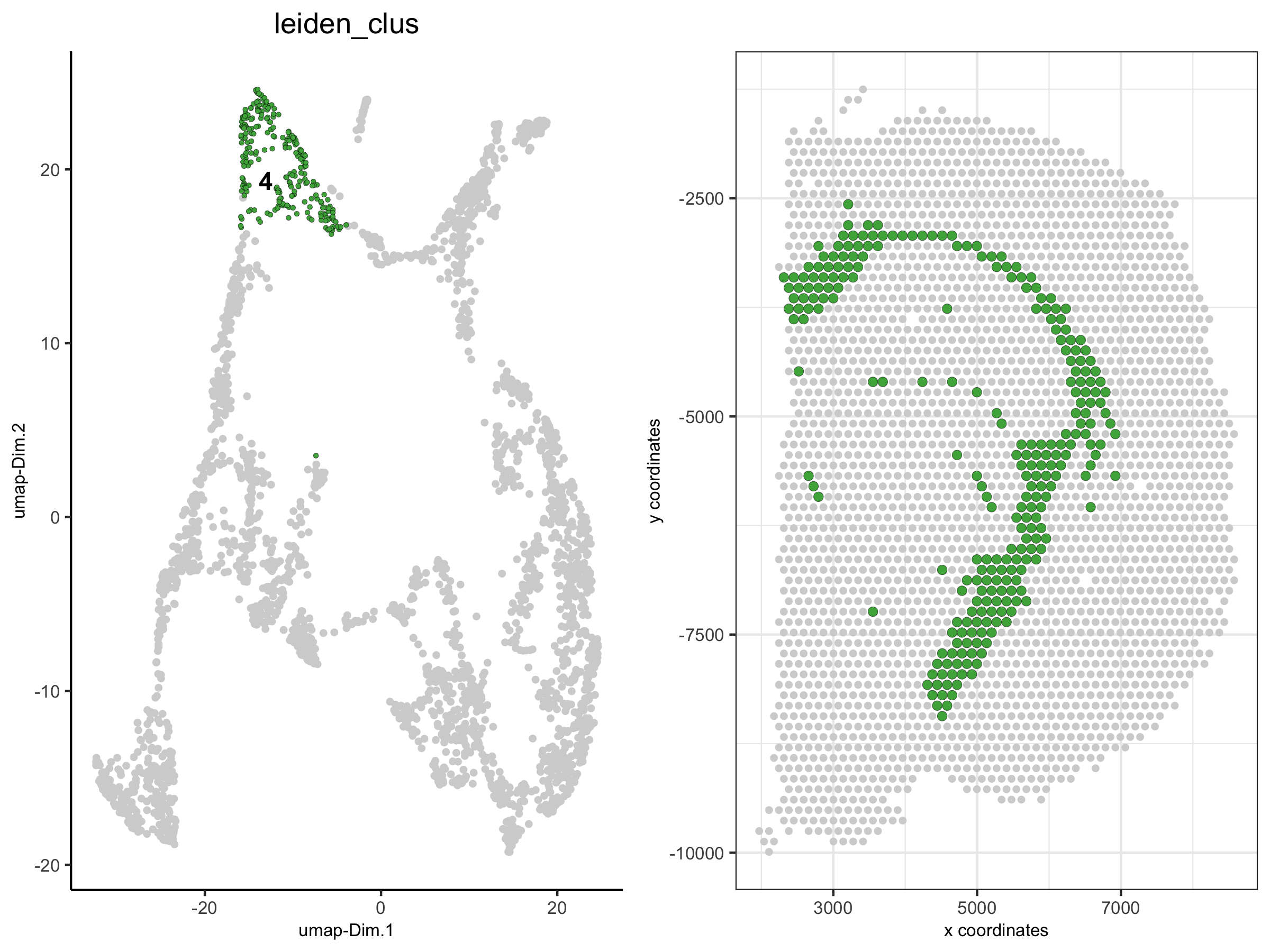## 3. Show Only A Subset of Clusters (excluding not selected cells)¶

```spatDimPlot(visium_brain,
cell_color = 'leiden_clus',  select_cell_groups = '4', show_other_cells = F,
plot_alignment = 'horizontal', spat_point_size = 2)
```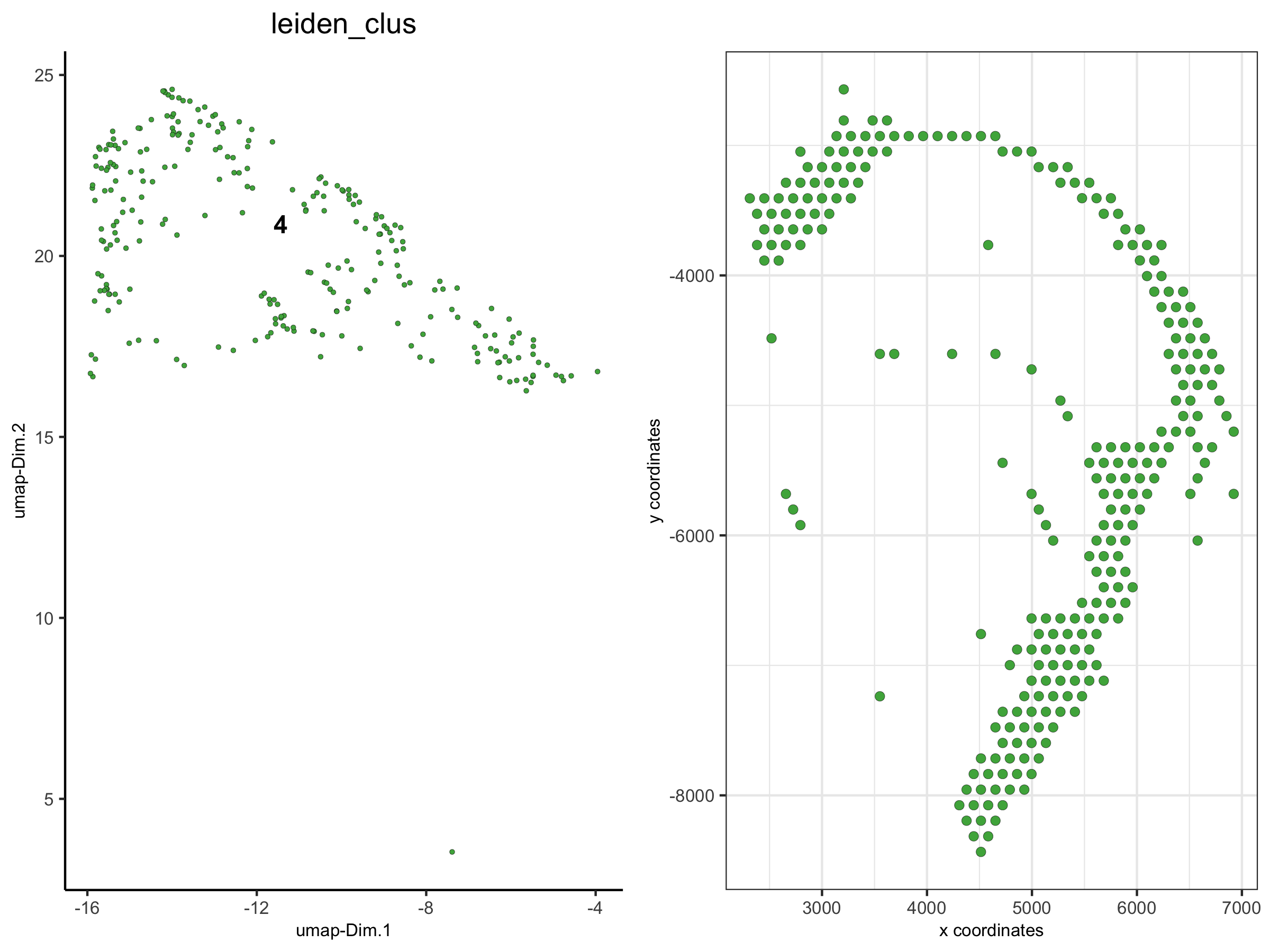## 4. Create Spatial Plots¶

These spatial plots have been grouped by the Leiden Clustering results

```spatPlot(visium_brain,
group_by = 'leiden_clus', group_by_subset = c(1:8),
cell_color = 'leiden_clus', cow_n_col = 4,
point_size = 1, axis_text = 6, axis_title = 6, legend_text = 6)
```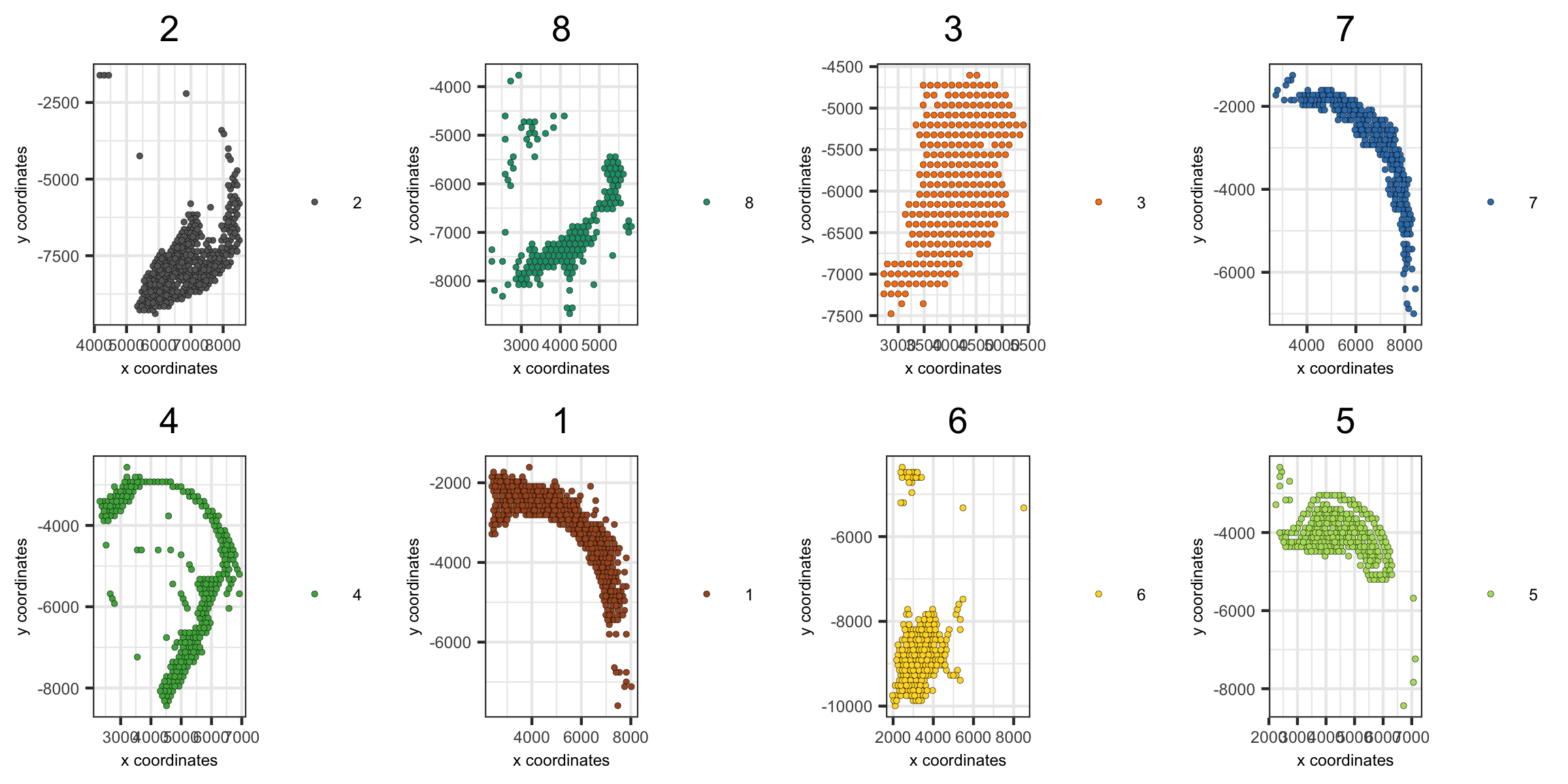```spatPlot(visium_brain,
group_by = 'leiden_clus', group_by_subset = c(9:16),
cell_color = 'leiden_clus', cow_n_col = 4,
point_size = 1, axis_text = 6, axis_title = 6, legend_text = 6)
```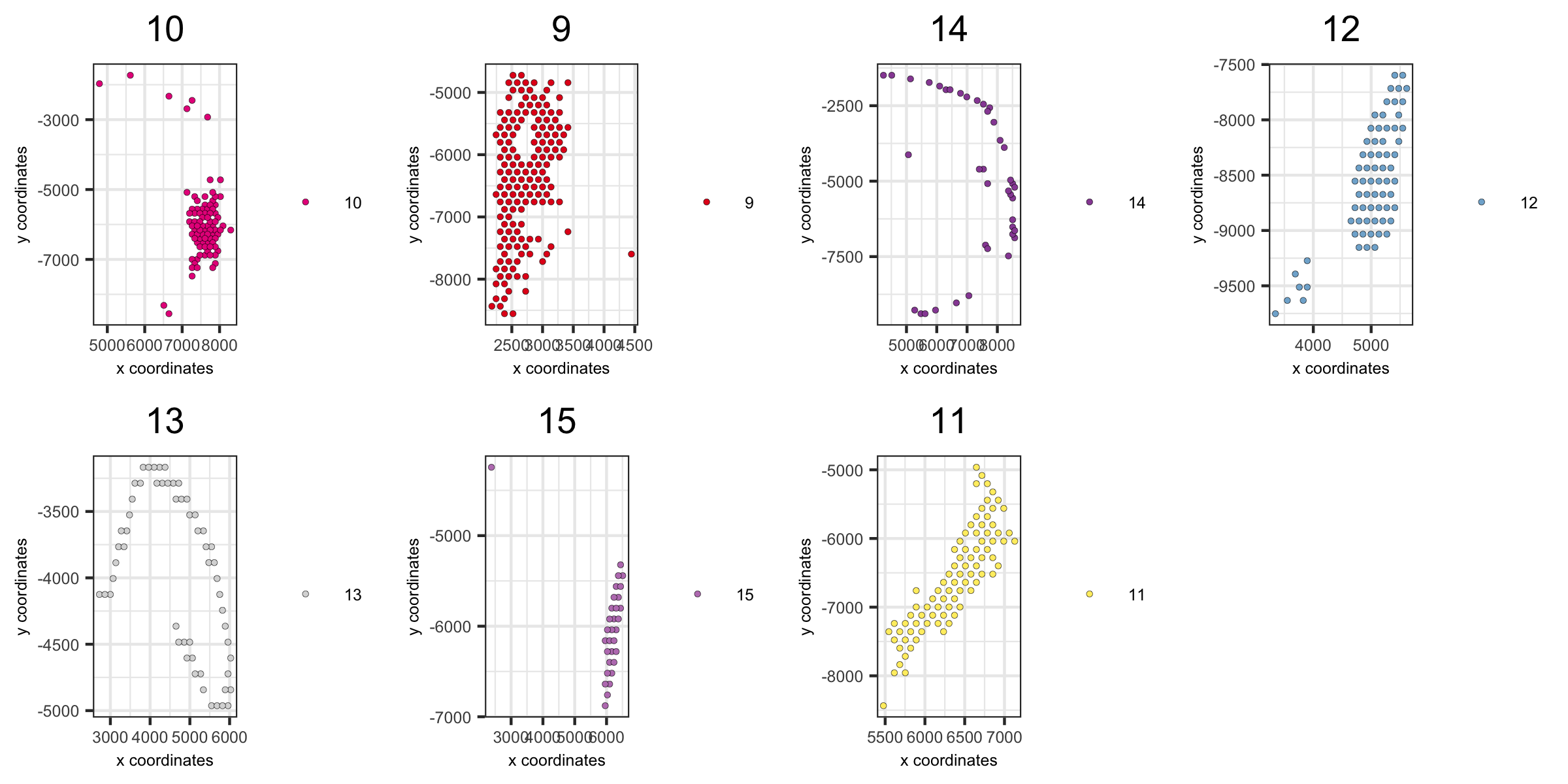## 5. Create Dimension Plots¶

These dimension plots have been grouped by the HMRF results.

```spatDimPlot(visium_brain, cell_color = 'HMRF_k12_b.1',
plot_alignment = 'horizontal', spat_point_size = 2)
```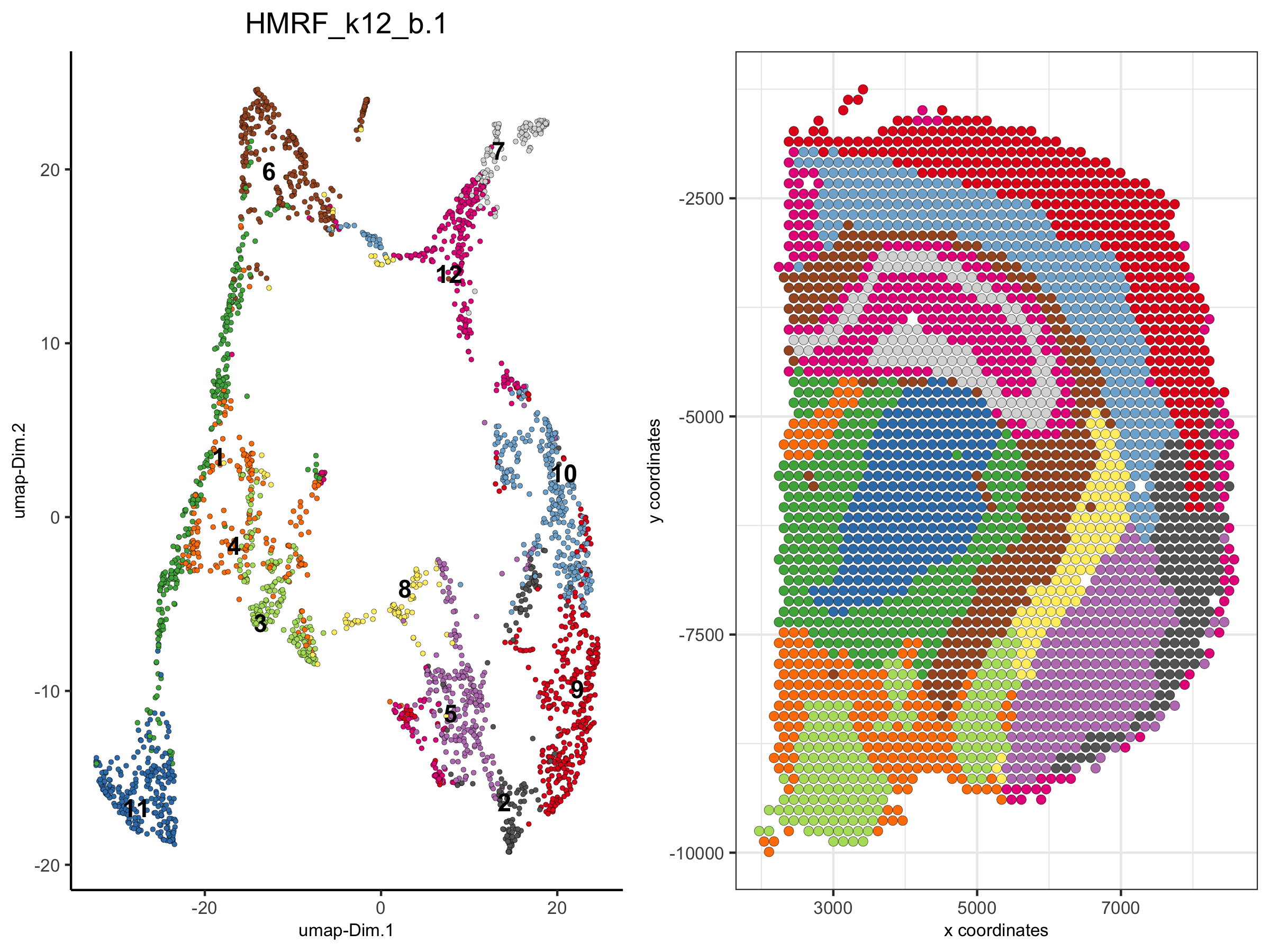```dimPlot(visium_brain,
group_by = 'HMRF_k12_b.1', group_by_subset = c(1:6),
cell_color = 'leiden_clus', cow_n_col = 3,
point_size = 1, axis_text = 6, axis_title = 6, legend_text = 6)
```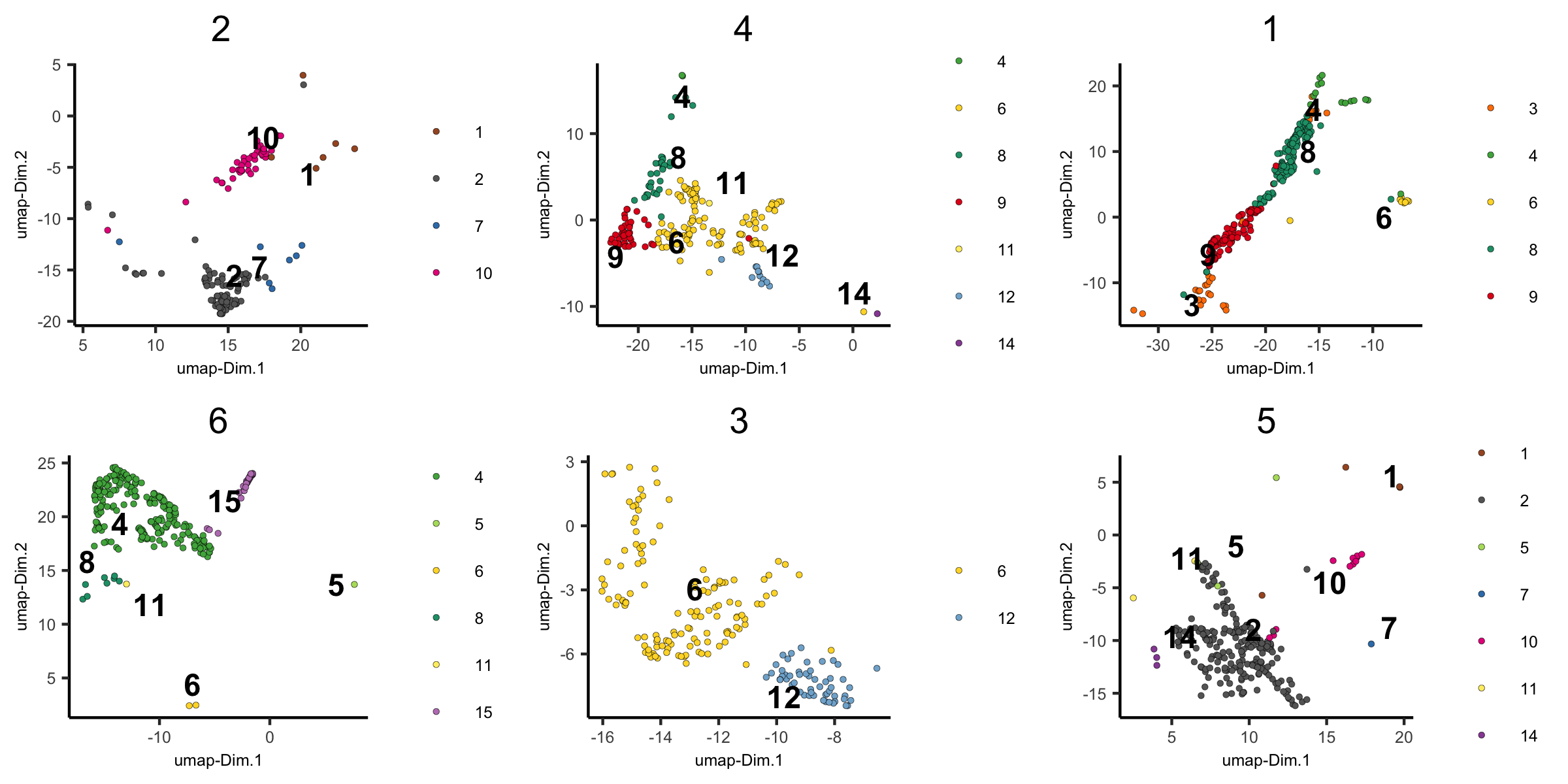```dimPlot(visium_brain,
group_by = 'HMRF_k12_b.1', group_by_subset = c(7:14),
cell_color = 'leiden_clus', cow_n_col = 3,
point_size = 1, axis_text = 6, axis_title = 6, legend_text = 6)
```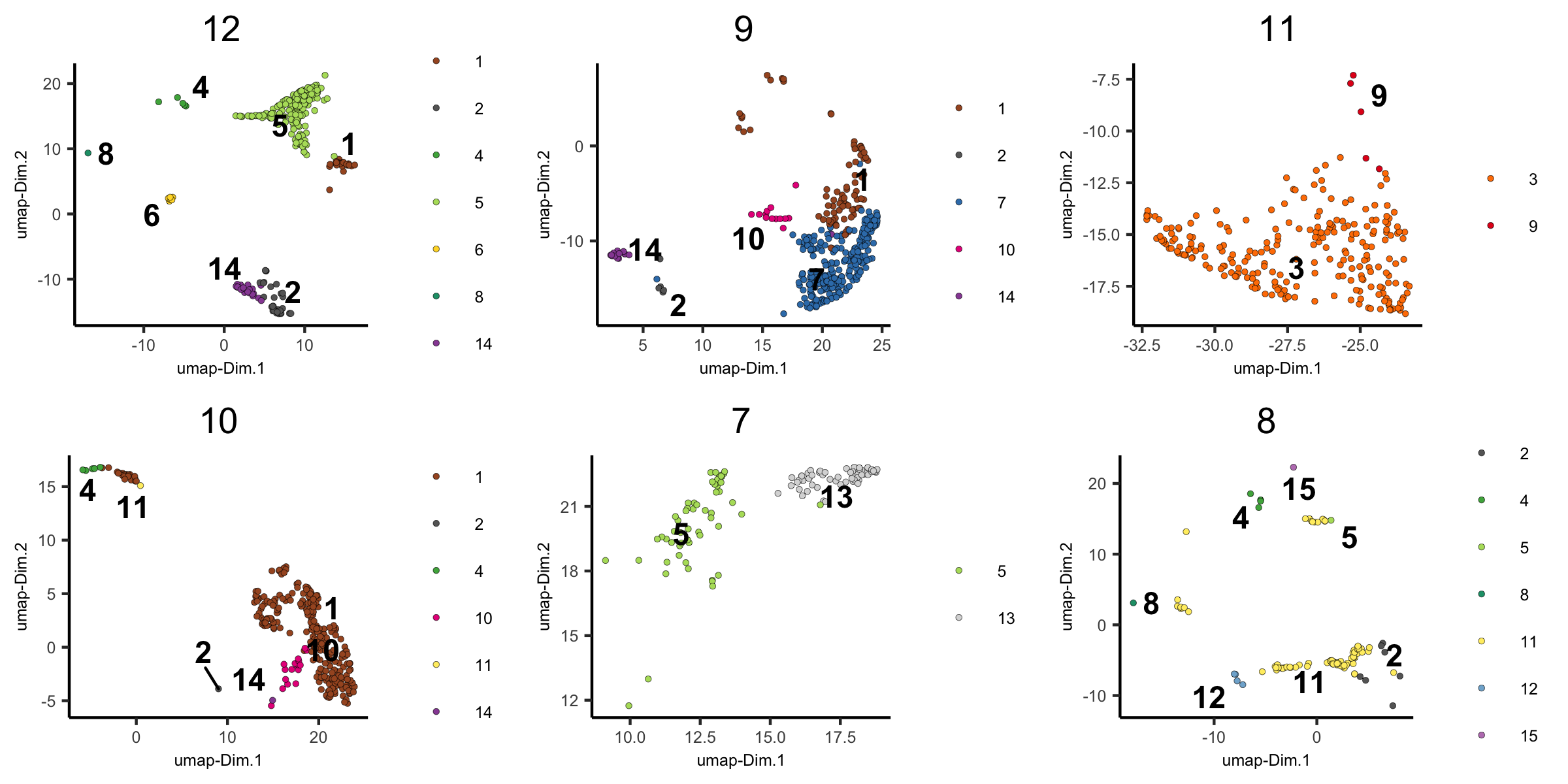## 6. Create A New Giotto Object By Subsetting Selected Clusters¶

```metadata = pDataDT(visium_brain)
subset_cell_IDs = metadata[leiden_clus %in% c(4, 5, 8, 13)]\$cell_ID
visium_brain_small = subsetGiotto(visium_brain, cell_ids = subset_cell_IDs)

spatDimPlot(visium_brain_small,
cell_color = 'leiden_clus',
plot_alignment = 'horizontal', spat_point_size = 2.5)
```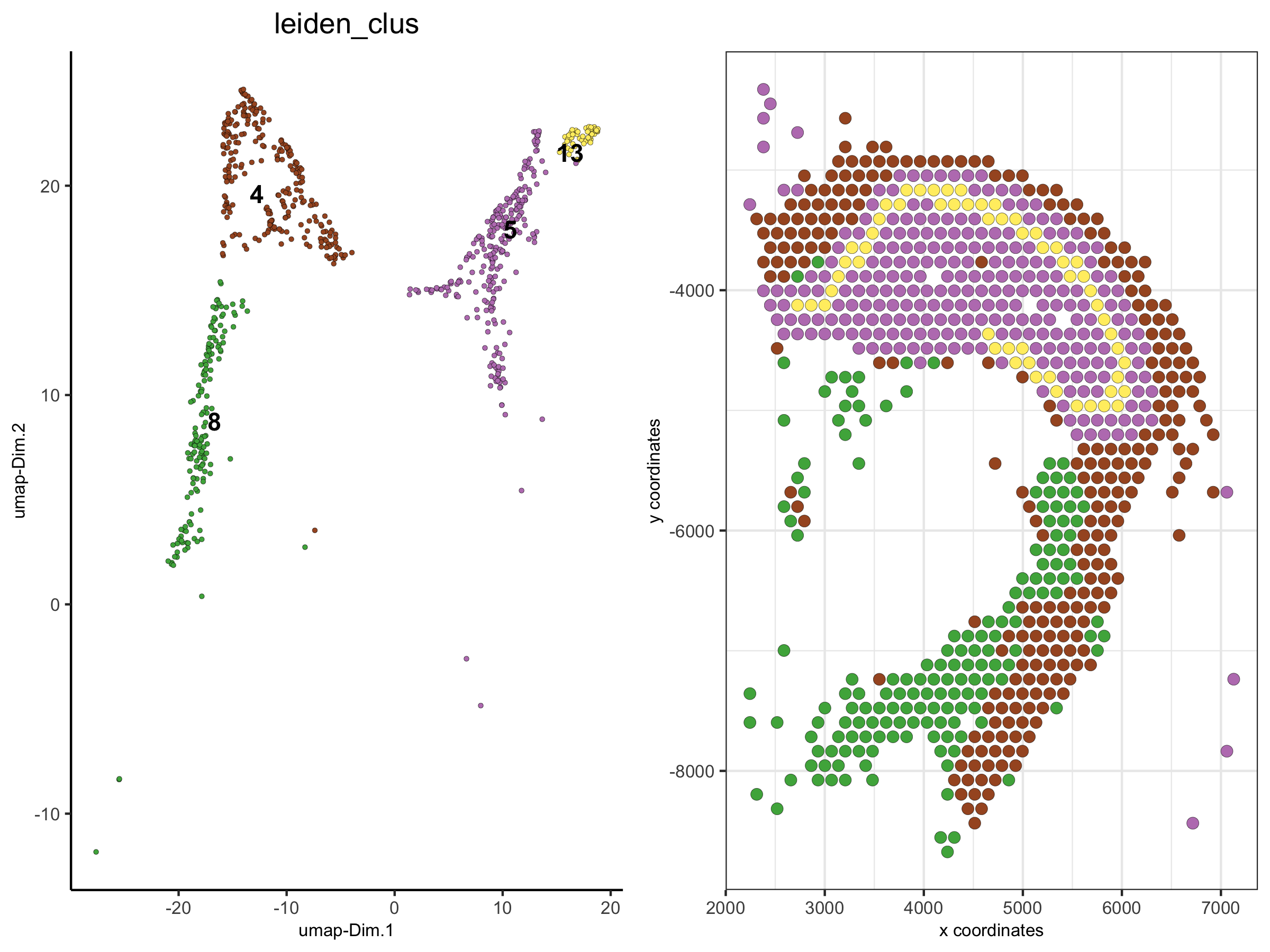```spatDimPlot(visium_brain_small,
cell_color = 'nr_genes', color_as_factor = F,
plot_alignment = 'horizontal', spat_point_size = 2.5)
```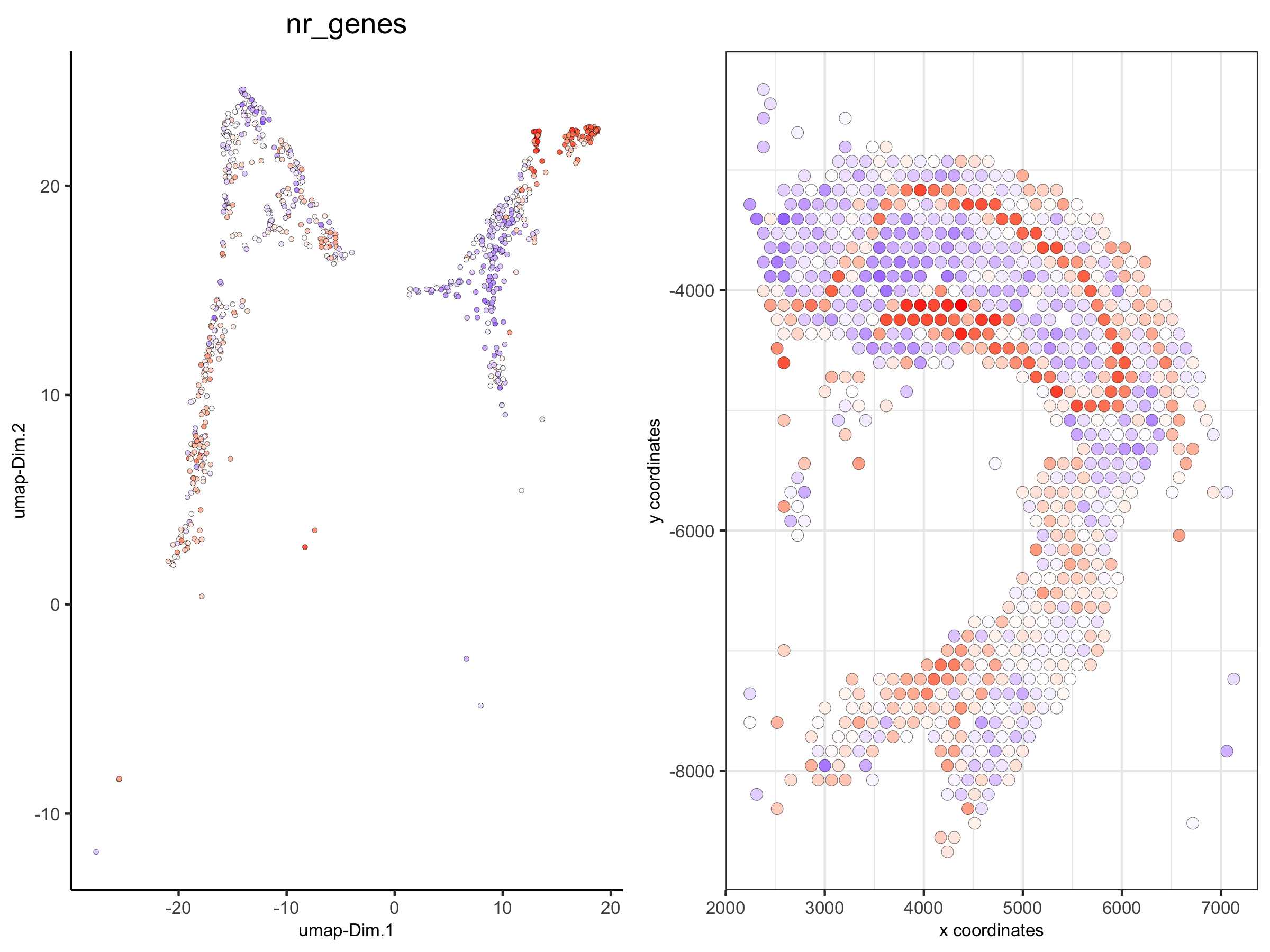## 7. Create A New Giotto Object By Subsetting Spatial Coordinates/Locations¶

```DG_subset = subsetGiottoLocs(visium_brain,
x_max = 6500, x_min = 3000,
y_max = -2500, y_min = -5500)

spatDimPlot(DG_subset,
cell_color = 'leiden_clus',
plot_alignment = 'horizontal', spat_point_size = 4,
save_param = list(save_name = '6_DG_loc_subset', base_height = 4))
```